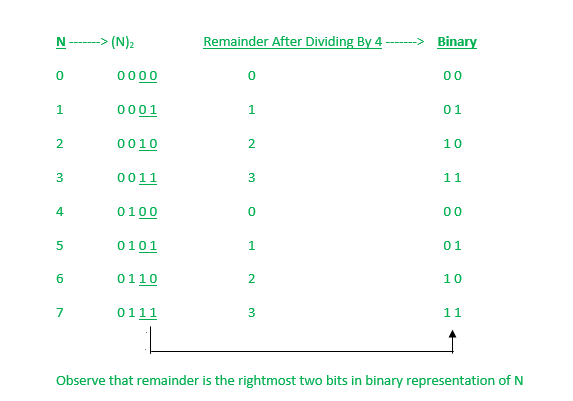Open in App
Not now

# Find the remainder when N is divided by 4 using Bitwise AND operator

• Difficulty Level : Basic
• Last Updated : 29 Mar, 2023

Given a number N, the task is to find the remainder when N is divided by 4 using Bitwise AND operator.

Examples:

Input: N = 98
Output: 2
Explanation: 98 % 4 = 2. Hence the output is 2.

Input: 200
Output: 0
Explanation: 200 % 4 = 0. Hence output is 0.

Naive approach:
For solving the above-mentioned problem we can use a naive method by using the Modulo (%) operator to find the remainder. But, the Modulo operator is computationally expensive and the method is inefficient.

Efficient Approach:
If we carefully observe the binary representation of N and its remainder with 4, we observe that remainder is simply the rightmost two bits in N. To get the rightmost two bits in number N, we perform bitwise AND (&) with 3 because 3 in binary is 0011. To understand the approach better let us have a look at the image below:Below is the implementation of the above approach:

## C

 `// C implementation to find N``// modulo 4 using Bitwise AND operator` `#include ` `// Function to find the remainder``int` `findRemainder(``int` `n)``{` `    ``// Bitwise AND with 3``    ``int` `x = n & 3;` `    ``// return  x``    ``return` `x;``}` `// Driver code``int` `main()``{` `    ``int` `N = 43;``    ``int` `ans = findRemainder(N);` `    ``printf``(``"%d"``, ans);` `    ``return` `0;``}`

## C++

 `// C++ implementation to find N``// modulo 4 using Bitwise AND operator` `#include ``using` `namespace` `std;` `// Function to find the remainder``int` `findRemainder(``int` `n)``{``    ``// Bitwise AND with 3``    ``int` `x = n & 3;` `    ``// Return  x``    ``return` `x;``}` `// Driver code``int` `main()``{` `    ``int` `N = 43;` `    ``int` `ans = findRemainder(N);` `    ``cout << ans << endl;` `    ``return` `0;``}`

## Java

 `// Java implementation to find N``// modulo 4 using Bitwise AND operator` `class` `Main {` `    ``// Driver code``    ``public` `static` `void` `main(String[] args)``    ``{` `        ``int` `N = ``43``;` `        ``int` `ans = findRemainder(N);` `        ``System.out.println(ans);``    ``}` `    ``// Function to find the remainder``    ``public` `static` `int` `findRemainder(``int` `n)``    ``{``        ``// Bitwise AND with 3``        ``int` `x = n & ``3``;` `        ``// return  x``        ``return` `x;``    ``}``}`

## Python 3

 `# Python 3 implementation to find N``# modulo 4 using Bitwise AND operator` `# Function to find the remainder``def` `findRemainder(n):``    ``# Bitwise AND with 3``    ``x ``=` `n & ``3` `    ``# Return  x``    ``return` `x` `# Driver code``if` `__name__ ``=``=` `'__main__'``:``    ``N ``=` `43` `    ``ans ``=` `findRemainder(N)` `    ``print``(ans)``    ` `# This code is contributed by Surendra_Gangwar`

## C#

 `// C# implementation to find N``// modulo 4 using Bitwise AND operator``using` `System;`` ` `class` `GFG {`` ` `    ``// Driver code``    ``public` `static` `void` `Main()``    ``{`` ` `        ``int` `N = 43;`` ` `        ``int` `ans = findRemainder(N);`` ` `        ``Console.Write(ans);``    ``}`` ` `    ``// Function to find the remainder``    ``public` `static` `int` `findRemainder(``int` `n)``    ``{``        ``// Bitwise AND with 3``        ``int` `x = n & 3;`` ` `        ``// return  x``        ``return` `x;``    ``}``}` `# This code is contributed by chitranayal`

## Javascript

 ``

Output

`3`

Time Complexity: O(1)
Auxiliary Space: O(1)

Another Approach:

We can solve this problem using  right shift operator (>>). The right shift operator shifts the bits of a number to the right by a specified number of positions. When we shift a number to the right by 2 positions (i.e., n >> 2), we effectively divide it by 4 and get the quotient as the result.

If we multiply the quotient by 4 and subtract it from the original number, we get the remainder. This can be expressed mathematically as:

N = 4 * (N >> 2) + (N & 3)

Below is the implementation of the above approach:

## C++

 `// C++ implementation to find N``// modulo 4 using Bitwise AND operator` `#include ``using` `namespace` `std;` `// Function to find the remainder``int` `findRemainder(``int` `n)``{``    ``// find quotient using right shift operator``    ``int` `quotient = n >> 2;``    ` `    ``// find remainder using bitwise AND operator``    ``int` `remainder = n - (quotient << 2);``    ` `    ``return` `remainder;``}`  `// Driver code``int` `main()``{``    ``int` `N = 43;``    ``int` `ans = findRemainder(N);``    ``cout << ans << endl;` `    ``return` `0;``}`

## Java

 `public` `class` `Main {``    ``// Function to find the remainder``    ``public` `static` `int` `findRemainder(``int` `n)``    ``{``        ``// find quotient using right shift operator``        ``int` `quotient = n >> ``2``;` `        ``// find remainder using bitwise AND operator``        ``int` `remainder = n - (quotient << ``2``);` `        ``return` `remainder;``    ``}` `    ``// Driver code``    ``public` `static` `void` `main(String[] args)``    ``{``        ``int` `N = ``43``;``        ``int` `ans = findRemainder(N);``        ``System.out.println(ans);``    ``}``}``// This code is contributed by Prajwal Kandekar`

Output

`3`

Time Complexity: O(1)

Auxiliary Space: O(1)

My Personal Notes arrow_drop_up